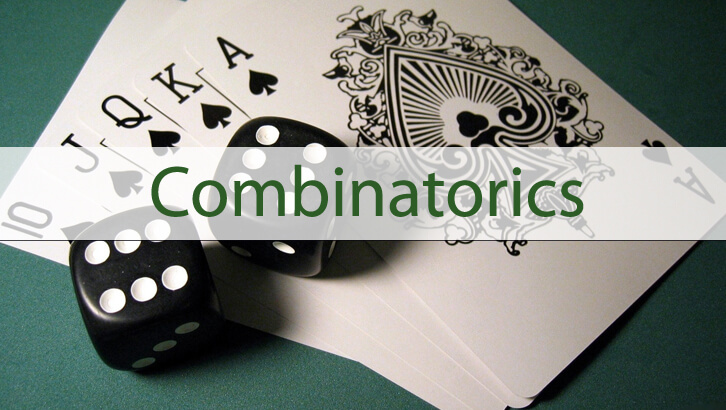# CAT Counting: Question with digits

This is an interesting question from Counting. Simple framework, but one needs to be very careful with the enumeration. One can get wrong answers in a number of ways.
Question
If we listed all numbers from 100 to 10,000, how many times would the digit 3 be printed?
A.      3980
B.      3700
C.      3840
D.      3780
Choice A
We need to consider all three digit and all 4-digit numbers.
Three-digit numbers: A B C. 3 can be printed in the 100’s place or10’s place or units place.
Ø  100’s place:  3 B C. B can take values 0 to 9, C can take values 0 to 9. So, 3 gets printed in the 100’s place 100 times
Ø  10’s place:  A 3 C. A can take values 1 to 9, C can take values 0 to 9. So, 3 gets printed in the 10’s place 90 times
Ø  Unit’s place:  A B 3. A can take values 1 to 9, B can take values 0 to 9. So, 3 gets printed in the unit’s place 90 times
So, 3 gets printed 280 times in 3-digit numbers
Four-digit numbers: A B C D. 3 can be printed in the 1000’s place, 100’s place or10’s place or units place.
Ø  1000’s place:  3 B C D. B can take values 0 to 9, C can take values 0 to 9, D can take values 0 to 9. So, 3 gets printed in the 100’s place 1000 times.
Ø  100’s place:  A 3 C D. A can take values 1 to 9, C & D can take values 0 to 9. So, 3 gets printed in the 100’s place 900 times.
Ø  10’s place:  A B 3 D. A can take values 1 to 9, B & D can take values 0 to 9. So, 3 gets printed in the 10’s place 900 times.
Ø  Unit’s place:  A B C 3. A can take values 1 to 9, B & C can take values 0 to 9. So, 3 gets printed in the unit’s place 900 times.
3 gets printed 3700 times in 4-digit numbers.
So, there are totally 3700 + 280 = 3980 numbers
1.Sam SID BID says: